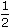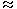# Aptitude - Time and Work

### Exercise :: Time and Work - General Questions

6.

If 6 men and 8 boys can do a piece of work in 10 days while 26 men and 48 boys can do the same in 2 days, the time taken by 15 men and 20 boys in doing the same type of work will be:

 A. 4 days B. 5 days C. 6 days D. 7 days

Explanation:

Let 1 man's 1 day's work = x and 1 boy's 1 day's work = y.

 Then, 6x + 8y = 1 and 26x + 48y = 1 . 10 2

 Solving these two equations, we get : x = 1 and y = 1 . 100 200

 (15 men + 20 boy)'s 1 day's work =15 + 20= 1 . 100 200 415 men and 20 boys can do the work in 4 days.

7.

A can do a piece of work in 4 hours; B and C together can do it in 3 hours, while A and C together can do it in 2 hours. How long will B alone take to do it?

 A. 8 hours B. 10 hours C. 12 hours D. 24 hours

Explanation:

 A's 1 hour's work = 1 ; 4

 (B + C)'s 1 hour's work = 1 ; 3

 (A + C)'s 1 hour's work = 1 . 2

 (A + B + C)'s 1 hour's work =1 + 1= 7 . 4 3 12

 B's 1 hour's work =7 - 1= 1 . 12 2 12B alone will take 12 hours to do the work.

8.

A can do a certain work in the same time in which B and C together can do it. If A and B together could do it in 10 days and C alone in 50 days, then B alone could do it in:

 A. 15 days B. 20 days C. 25 days D. 30 days

Explanation:

 (A + B)'s 1 day's work = 1 10

 C's 1 day's work = 1 50

 (A + B + C)'s 1 day's work =1 + 1= 6 = 3 . .... (i) 10 50 50 25

A's 1 day's work = (B + C)'s 1 day's work .... (ii)

 From (i) and (ii), we get: 2 x (A's 1 day's work) = 3 25A's 1 day's work = 3 . 50B's 1 day's work1 - 3= 2 = 1 . 10 50 50 25

So, B alone could do the work in 25 days.

9.

A does 80% of a work in 20 days. He then calls in B and they together finish the remaining work in 3 days. How long B alone would take to do the whole work?

 A. 23 days B. 37 days C. 37D. 40 days

Explanation:

 Whole work is done by A in20 x 5= 25 days. 4

 Now,1 - 4i.e., 1 work is done by A and B in 3 days. 5 5

Whole work will be done by A and B in (3 x 5) = 15 days.

 A's 1 day's work = 1 , (A + B)'s 1 day's work = 1 . 25 15B's 1 day's work =1 - 1= 4 = 2 . 15 25 150 75

 So, B alone would do the work in 75 = 37 1 days. 2 2

10.

A machine P can print one lakh books in 8 hours, machine Q can print the same number of books in 10 hours while machine R can print them in 12 hours. All the machines are started at 9 A.M. while machine P is closed at 11 A.M. and the remaining two machines complete work. Approximately at what time will the work (to print one lakh books) be finished ?

 A. 11:30 A.M. B. 12 noon C. 12:30 P.M. D. 1:00 P.M.

Explanation:

 (P + Q + R)'s 1 hour's work =1 + 1 + 1= 37 . 8 10 12 120

 Work done by P, Q and R in 2 hours =37 x 2= 37 . 120 60

 Remaining work =1 - 37= 23 . 60 60

 (Q + R)'s 1 hour's work =1 + 1= 11 . 10 12 60

 Now, 11 work is done by Q and R in 1 hour. 60

 So, 23 work will be done by Q and R in60 x 23= 23 hours2 hours. 60 11 60 11

So, the work will be finished approximately 2 hours after 11 A.M., i.e., around 1 P.M.